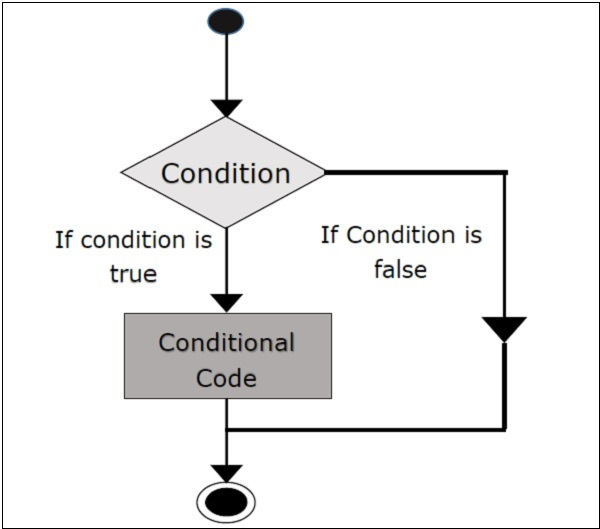# Scala - IF ELSE Statements

This chapter takes you through the conditional construction statements in Scala programming. Following is the general form of a typical decision making IF...ELSE structure found in most of the programming languages.

## Flow Chart

The following is a flow chart diagram for conditional statement.## if Statement

‘if’ statement consists of a Boolean expression followed by one or more statements.

### Syntax

The syntax of an ‘if’ statement is as follows.

```if(Boolean_expression) {
// Statements will execute if the Boolean expression is true
}
```

If the Boolean expression evaluates to true then the block of code inside the ‘if’ expression will be executed. If not, the first set of code after the end of the ‘if’ expression (after the closing curly brace) will be executed.

Try the following example program to understand conditional expressions (if expression) in Scala Programming Language.

### Example

```object Demo {
def main(args: Array[String]) {
var x = 10;

if( x < 20 ){
println("This is if statement");
}
}
}
```

Save the above program in Demo.scala. The following commands are used to compile and execute this program.

### Command

```\>scalac Demo.scala
\>scala Demo
```

### Output

```This is if statement
```

## If-else Statement

An ‘if’ statement can be followed by an optional else statement, which executes when the Boolean expression is false.

### Syntax

The syntax of a if...else is −

```if(Boolean_expression){
//Executes when the Boolean expression is true
} else{
//Executes when the Boolean expression is false
}
```

Try the following example program to understand conditional statements (if- else statement) in Scala Programming Language.

### Example

```object Demo {
def main(args: Array[String]) {
var x = 30;

if( x < 20 ){
println("This is if statement");
} else {
println("This is else statement");
}
}
}
```

Save the above program in Demo.scala. The following commands are used to compile and execute this program.

### Command

```\>scalac Demo.scala
\>scala Demo
```

### Output

```This is else statement
```

## If-else-if-else Statement

An 'if' statement can be followed by an optional 'else if...else' statement, which is very useful to test various conditions using single if...else if statement.

When using if , else if , else statements there are few points to keep in mind.

• An 'if' can have zero or one else's and it must come after any else if's.

• An 'if' can have zero to many else if's and they must come before the else.

• Once an else if succeeds, none of he remaining else if's or else's will be tested.

### Syntax

The following is the syntax of an ‘if...else if...else’ is as follows −

```if(Boolean_expression 1){
//Executes when the Boolean expression 1 is true
} else if(Boolean_expression 2){
//Executes when the Boolean expression 2 is true
} else if(Boolean_expression 3){
//Executes when the Boolean expression 3 is true
} else {
//Executes when the none of the above condition is true.
}
```

Try the following example program to understand conditional statements (if- else- if- else statement) in Scala Programming Language.

### Example

```object Demo {
def main(args: Array[String]) {
var x = 30;

if( x == 10 ){
println("Value of X is 10");
} else if( x == 20 ){
println("Value of X is 20");
} else if( x == 30 ){
println("Value of X is 30");
} else{
println("This is else statement");
}
}
}
```

Save the above program in Demo.scala. The following commands are used to compile and execute this program.

### Command

```\>scalac Demo.scala
\>scala Demo
```

### Output

```Value of X is 30
```

## Nested if-else Statement

It is always legal to nest if-else statements, which means you can use one if or else-if statement inside another if or else-if statement.

### Syntax

The syntax for a nested if-else is as follows −

```if(Boolean_expression 1){
//Executes when the Boolean expression 1 is true

if(Boolean_expression 2){
//Executes when the Boolean expression 2 is true
}
}
```

Try the following example program to understand conditional statements (nested- if statement) in Scala Programming Language.

### Example

```object Demo {
def main(args: Array[String]) {
var x = 30;
var y = 10;

if( x == 30 ){
if( y == 10 ){
println("X = 30 and Y = 10");
}
}
}
}
```

Save the above program in Demo.scala. The following commands are used to compile and execute this program.

### Command

```\>scalac Demo.scala
\>scala Demo
```

### Output

```X = 30 and Y = 10
```